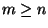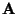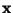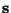Next: Relations to classical methods Up: Independent Component Analysis Previous: Definitions of linear independent

## Identifiability of the ICA model

The identifiability of the noise-free ICA model has been treated in . By imposing the following fundamental restrictions (in addition to the basic assumption of statistical independence), the identifiability of the model can be assured.
1.
All the independent components si, with the possible exception of one component, must be non-Gaussian.
2.
The number of observed linear mixtures m must be at least as large as the number of independent components n, i.e.,.
3.
The matrixmust be of full column rank.
Usually, it is also assumed thatandare centered, which is in practice no restriction, as this can always be accomplished by subtracting the mean from the random vector. Ifandare interpreted as stochastic processes instead of simply random variables, additional restrictions are necessary. At the minimum, one has to assume that the stochastic processes are stationary in the strict sense. Some restrictions of ergodicity with respect to the quantities estimated are also necessary . These assumptions are fulfilled, for example, if the process is i.i.d. over time. After such assumptions, one can consider the stochastic process as a random variable, as we do here.

A basic, but rather insignificant indeterminacy in the model is that the independent components and the columns ofcan only be estimated up to a multiplicative constant, because any constant multiplying an independent component in Eq. (11) could be canceled by dividing the corresponding column of the mixing matrixby the same constant. For mathematical convenience, one usually defines that the independent components si have unit variance. This makes the independent components unique, up to a multiplicative sign (which may be different for each component) .

The definitions of ICA given above imply no ordering of the independent components, which is in contrast to, e.g., PCA. It is possible, however, to introduce an order between the independent components. One way is to use the norms of the columns of the mixing matrix, which give the contributions of the independent components to the variances of the xi. Ordering the si according to descending norm of the corresponding columns of, for example, gives an ordering reminiscent of PCA. A second way is to use the non-Gaussianity of the independent components. Non-Gaussianity may be measured, for example, using one of the projection pursuit indexes in Section 2.3.1 or the contrast functions to be introduced in Section 4.4.3. Ordering the si according to non-Gaussianity gives an ordering related to projection pursuit.

The first restriction (non-Gaussianity) in the list above, is necessary for the identifiability of the ICA model . Indeed, for Gaussian random variables mere uncorrelatedness implies independence, and thus any decorrelating representation would give independent components. Nevertheless, if more than one of the components si are Gaussian, it is still possible to identify the non-Gaussian independent components, as well as the corresponding columns of the mixing matrix.

On the other hand, the second restriction,, is not completely necessary. Even in the case where m<n, the mixing matrixseems to be identifiable  (though no rigorous proofs exist to our knowledge), whereas the realizations of the independent components are not identifiable, because of the noninvertibility of. However, most of the existing theory for ICA is not valid in this case, and therefore we have to make the second assumption in this paper. Recent work on the case m<n, often called ICA with overcomplete bases, can be found in [21,118,98,99,69].

Some rank restriction on the mixing matrix, like the third restriction given above, is also necessary, though the form given here is probably not the weakest possible.

As regards the identifiability of the noisy ICA model, the same three restrictions seem to guarantee partial identifiability, if the noise is assumed to be independent from the components si [35,93,107]. In fact, the noisy ICA model is a special case of the noise-free ICA model with m<n, because the noise variables could be considered as additional independent components. In particular, the mixing matrixis still identifiable. In contrast, the realizations of the independent components si can no longer be identified, because they cannot be completely separated from noise. It would seem that the noise covariance matrix is also identifiable .

In this paper, we shall assume that the assumptions 1-3 announced above are valid, and we shall treat only the noiseless ICA model, except for some comments on the estimation of the noisy model. We also make the conventional assumption that the dimension of the observed data equals the number of the independent components, i.e., n=m. This simplification is justified the fact that if m>n, the dimension of the observed vector can always be reduced so that m=n. Such a reduction of dimension can be achieved by existing methods such as PCA.Next: Relations to classical methods Up: Independent Component Analysis Previous: Definitions of linear independent
Aapo Hyvarinen
1999-04-23